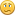## PIC 32 MAXI WEB_declaration of input and output ports

Started by ALFREDOSKY, July 11, 2014, 02:31:31 AM

#### ALFREDOSKY

Hi all, i saw the code from demo and i found this in BSP.h file

`// DIGITAL INS#define DIGITAL_IN_1 (1 << 7)#define DIGITAL_IN_2 (1 << 6)// buttons#define BUTTON_1 (1 << 14)#define BUTTON_2 (1 << 15)#define BUTTON_3 (1 << 13)`

was the first time i saw that, please somebody explain me how works those lines

#### Stanimir5F

#1
Those are just mask macros for the buttons and digital inputs. You can see in the respective BSP.c file how are they used.
May the Source be with You!

#### ALFREDOSKY

#2
Yes Stanimir5F, i know it but i do not understand the syntax (1 << 7) or (1 << 15), i am not sure if it refers a specific number port and what port is...that's my doubt!#### JohnS

#3
Grab any book on C or read online.

<< is left shift, >> right.

John

#### Stanimir5F

#4
The macro itself isn't associated with any port. It is just a value. If the shift operator (<<) is what confuses you can read about it here: http://en.wikipedia.org/wiki/Bitwise_operation or http://www.cprogramming.com/tutorial/bitwise_operators.html
So in this case when you define:
`#define DIGITAL_IN_1 (1 << 7)`
it is the same like
`#define DIGITAL_IN_1 (0x80)`
since
1<<0 = 1 (binary) = 0x01 (hexadecimal)
1<<1 = 10 (binary) = 0x02 (hexadecimal)
1<<2 = 100 (binary) = 0x04 (hexadecimal)
1<<3 = 1000 (binary) = 0x08 (hexadecimal)
1<<4 = 10000 (binary) = 0x10 (hexadecimal)
1<<5 = 100000 (binary) = 0x20 (hexadecimal)
1<<6 = 1000000 (binary) = 0x40 (hexadecimal)
1<<7 = 10000000 (binary) = 0x80 (hexadecimal)

You can see where it (the macro) is used by searching in the project. You will find this place (BSP.c, line 297-298):
` tmp = PORTRead(IOPORT_G); tmp &= DIGITAL_IN_1 | DIGITAL_IN_2;`
Here first we scan the whole PORTG value and then get only the values we need masking it with the defined macros and bitwise "AND". But once again - the macro itself IS NOT associated with a specific port or address or whatever. After all it is just a text (and in this case the text is a number).

It is similar with the BUTTON_x macros. But since their pins on the PIC are multiplexed with LCD they can't be scanned as GPIO. So their (macros) value isn't associated with a specific port function (like PORTD.13) but with PMD function (PMD13). That means the way they are scanned is different (check the definition of function "ButtonsUpdate" and where it is called).

Stanimir, Olimex
May the Source be with You!

#### ALFREDOSKY

#5
Hi Stanimir5F, thank you very much for your time, i understand now